# 5.4: Solve Applications with Systems of Equations

•• OpenStax
• OpenStax
$$\newcommand{\vecs}{\overset { \rightharpoonup} {\mathbf{#1}} }$$ $$\newcommand{\vecd}{\overset{-\!-\!\rightharpoonup}{\vphantom{a}\smash {#1}}}$$$$\newcommand{\id}{\mathrm{id}}$$ $$\newcommand{\Span}{\mathrm{span}}$$ $$\newcommand{\kernel}{\mathrm{null}\,}$$ $$\newcommand{\range}{\mathrm{range}\,}$$ $$\newcommand{\RealPart}{\mathrm{Re}}$$ $$\newcommand{\ImaginaryPart}{\mathrm{Im}}$$ $$\newcommand{\Argument}{\mathrm{Arg}}$$ $$\newcommand{\norm}{\| #1 \|}$$ $$\newcommand{\inner}{\langle #1, #2 \rangle}$$ $$\newcommand{\Span}{\mathrm{span}}$$ $$\newcommand{\id}{\mathrm{id}}$$ $$\newcommand{\Span}{\mathrm{span}}$$ $$\newcommand{\kernel}{\mathrm{null}\,}$$ $$\newcommand{\range}{\mathrm{range}\,}$$ $$\newcommand{\RealPart}{\mathrm{Re}}$$ $$\newcommand{\ImaginaryPart}{\mathrm{Im}}$$ $$\newcommand{\Argument}{\mathrm{Arg}}$$ $$\newcommand{\norm}{\| #1 \|}$$ $$\newcommand{\inner}{\langle #1, #2 \rangle}$$ $$\newcommand{\Span}{\mathrm{span}}$$$$\newcommand{\AA}{\unicode[.8,0]{x212B}}$$

##### Learning Objectives

By the end of this section, you will be able to:

• Translate to a system of equations
• Solve direct translation applications
• Solve geometry applications
• Solve uniform motion applications
##### Note

Before you get started, take this readiness quiz.

1. The sum of twice a number and nine is 31. Find the number.
If you missed this problem, review Exercise 3.1.10.
2. Twins Jon and Ron together earned $96,000 last year. Ron earned$8,000 more than three times what Jon earned. How much did each of the twins earn?
If you missed this problem, review Exercise 3.1.31.
3. Alessio rides his bike $$3\frac{1}{2}$$ hours at a rate of 10 miles per hour. How far did he ride?
If you missed this problem, review Exercise 2.6.1.

Previously in this chapter we solved several applications with systems of linear equations. In this section, we’ll look at some specific types of applications that relate two quantities. We’ll translate the words into linear equations, decide which is the most convenient method to use, and then solve them.

We will use our Problem Solving Strategy for Systems of Linear Equations.

USE A PROBLEM SOLVING STRATEGY FOR SYSTEMS OF LINEAR EQUATIONS.

1. Read the problem. Make sure all the words and ideas are understood.
2. Identify what we are looking for.
3. Name what we are looking for. Choose variables to represent those quantities.
4. Translate into a system of equations.
5. Solve the system of equations using good algebra techniques.
6. Check the answer in the problem and make sure it makes sense.
7. Answer the question with a complete sentence.

## Translate to a System of Equations

Many of the problems we solved in earlier applications related two quantities. Here are two of the examples from the chapter on Math Models.

• The sum of two numbers is negative fourteen. One number is four less than the other. Find the numbers.
• A married couple together earns $110,000 a year. The wife earns$16,000 less than twice what her husband earns. What does the husband earn?

In that chapter we translated each situation into one equation using only one variable. Sometimes it was a bit of a challenge figuring out how to name the two quantities, wasn’t it?

Let’s see how we can translate these two problems into a system of equations with two variables. We’ll focus on Steps 1 through 4 of our Problem Solving Strategy.

##### Exercise $$\PageIndex{1}$$: How to Translate to a System of Equations

Translate to a system of equations:

The sum of two numbers is negative fourteen. One number is four less than the other. Find the numbers.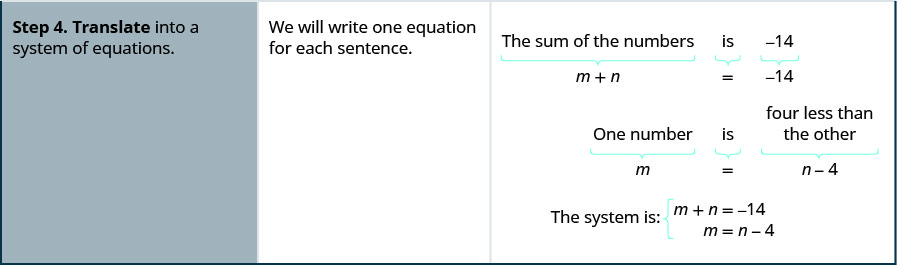##### Exercise $$\PageIndex{2}$$

Translate to a system of equations:

The sum of two numbers is negative twenty-three. One number is 7 less than the other. Find the numbers.

$$\left\{\begin{array}{l}{m+n=-23} \\ {m=n-7}\end{array}\right.$$

##### Exercise $$\PageIndex{3}$$

Translate to a system of equations:

The sum of two numbers is negative eighteen. One number is 40 more than the other. Find the numbers.

$$\left\{\begin{array}{l}{m+n=-18} \\ {m=n+40}\end{array}\right.$$

We’ll do another example where we stop after we write the system of equations.

##### Exercise $$\PageIndex{4}$$

Translate to a system of equations:

A married couple together earns $110,000 a year. The wife earns$16,000 less than twice what her husband earns. What does the husband earn?

$$\begin{array}{ll}{\text {We are looking for the amount that }} & {\text {Let } h=\text { the amount the husband earns. }} \\ {\text {the husband and wife each earn. }} & { w=\text { the amount the wife earns }} \\ {\text{Translate.}} & {\text{A married couple together earns \110,000.} }\\ {} & {w+h=110000} \\ & \text{The wife earns \16,000 less than twice what} \\ & \text{husband earns.} \\ & w=2h−16,000 \\ \text{The system of equations is:} & \left\{\begin{array}{l}{w+h=110,000} \\ {w=2 h-16,000}\end{array}\right.\end{array}$$

##### Exercise $$\PageIndex{5}$$

Translate to a system of equations:

A couple has a total household income of $84,000. The husband earns$18,000 less than twice what the wife earns. How much does the wife earn?

$$\left\{\begin{array}{l}{w+h=84,000} \\ {h=2 w-18,000}\end{array}\right.$$

##### Exercise $$\PageIndex{6}$$

Translate to a system of equations:

A senior employee makes $5 less than twice what a new employee makes per hour. Together they make$43 per hour. How much does each employee make per hour?

$$\left\{\begin{array}{l}{s=2 n-5} \\ {s+n=43}\end{array}\right.$$

## Solve Direct Translation Applications

We set up, but did not solve, the systems of equations in Exercise $$\PageIndex{1}$$ and Exercise $$\PageIndex{4}$$ Now we’ll translate a situation to a system of equations and then solve it.

##### Exercise $$\PageIndex{7}$$

Translate to a system of equations and then solve:

Devon is 26 years older than his son Cooper. The sum of their ages is 50. Find their ages.

 Step 1. Read the problem. Step 2. Identify what we are looking for. We are looking for the ages of Devon and Cooper. Step 3. Name what we are looking for. Let d= Devon’s age. c= Cooper’s age Step 4. Translate into a system of equations. Devon is 26 years older than Cooper.The sum of their ages is 50.The system is:Step 5. Solve the system of equations. Solve by substitution.Substitute c + 26 into the second equation.Solve for c.Substitute c = 12 into the first equation and then solve for d.Step 6. Check the answer in the problem. Is Devon’s age 26 more than Cooper’s? Yes, 38 is 26 more than 12. Is the sum of their ages 50? Yes, 38 plus 12 is 50. Step 7. Answer the question. Devon is 38 and Cooper is 12 years old.
##### Exercise $$\PageIndex{8}$$

Translate to a system of equations and then solve:

Ali is 12 years older than his youngest sister, Jameela. The sum of their ages is 40. Find their ages.

Ali is 26 and Jameela is 14.

##### Exercise $$\PageIndex{9}$$

Translate to a system of equations and then solve:

Jake’s dad is 6 more than 3 times Jake’s age. The sum of their ages is 42. Find their ages.

Jake is 9 and his dad is 33.

##### Exercise $$\PageIndex{10}$$

Translate to a system of equations and then solve:

When Jenna spent 10 minutes on the elliptical trainer and then did circuit training for 20 minutes, her fitness app says she burned 278 calories. When she spent 20 minutes on the elliptical trainer and 30 minutes circuit training she burned 473 calories. How many calories does she burn for each minute on the elliptical trainer? How many calories does she burn for each minute of circuit training?

 Step 1. Read the problem. Step 2. Identify what we are looking for. We are looking for the number of calories burned each minute on the elliptical trainer and each minute of circuit training. Step 3. Name what we are looking for. Let e= number of calories burned per minute on the elliptical trainer. c= number of calories burned per minute while circuit training Step 4. Translate into a system of equations. 10 minutes on the elliptical and circuit training for 20 minutes, burned 278 calories20 minutes on the elliptical and 30 minutes of circuit training burned 473 calories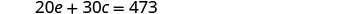The system is: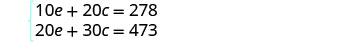Step 5. Solve the system of equations. Multiply the first equation by −2 to get opposite coefficients of e.Simplify and add the equations. Solve for c.Substitute c = 8.3 into one of the original equations to solve for e.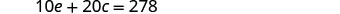Step 6. Check the answer in the problem. Check the math on your own.Step 7. Answer the question. Jenna burns 8.3 calories per minute circuit training and 11.2 calories per minute while on the elliptical trainer.
##### Exercise $$\PageIndex{11}$$

Translate to a system of equations and then solve:

Mark went to the gym and did 40 minutes of Bikram hot yoga and 10 minutes of jumping jacks. He burned 510 calories. The next time he went to the gym, he did 30 minutes of Bikram hot yoga and 20 minutes of jumping jacks burning 470 calories. How many calories were burned for each minute of yoga? How many calories were burned for each minute of jumping jacks?

Mark burned 11 calories for each minute of yoga and 7 calories for each minute of jumping jacks.

##### Exercise $$\PageIndex{12}$$

Translate to a system of equations and then solve:

Erin spent 30 minutes on the rowing machine and 20 minutes lifting weights at the gym and burned 430 calories. During her next visit to the gym she spent 50 minutes on the rowing machine and 10 minutes lifting weights and burned 600 calories. How many calories did she burn for each minutes on the rowing machine? How many calories did she burn for each minute of weight lifting?

Erin burned 11 calories for each minute on the rowing machine and 5 calories for each minute of weight lifting.

## Solve Geometry Applications

When we learned about Math Models, we solved geometry applications using properties of triangles and rectangles. Now we’ll add to our list some properties of angles.

The measures of two complementary angles add to 90 degrees. The measures of two supplementary angles add to 180 degrees.

##### COMPLEMENTARY AND SUPPLEMENTARY ANGLES

Two angles are complementary if the sum of the measures of their angles is 90 degrees.

Two angles are supplementary if the sum of the measures of their angles is 180 degrees.

If two angles are complementary, we say that one angle is the complement of the other.

If two angles are supplementary, we say that one angle is the supplement of the other.

##### Exercise $$\PageIndex{13}$$

Translate to a system of equations and then solve:

The difference of two complementary angles is 26 degrees. Find the measures of the angles.

$$\begin{array}{ll}{\textbf {Step 1. Read}\text{ the problem. }} & {} \\ {\textbf {Step 2. Identify}\text{ what we are looking for.}} & {\text {We are looking for the measure of each angle.}} \\ \\ {\textbf{Step 3. Name}\text{ what we are looking for.}} & {\text{Let x = the measure of the first angle.} }\\ {} & \text{y = the measure of the second angle} \\ \textbf{Step 4. Translate}\text{ into a system of equations.}& \text{The angles are complementary.} \\ & \text{x+y=90} \\ & \text{The difference of the two angles is 26 degrees.} \\ & \text{x−y=26} \\ \\ \text{The system is} & {\left\{\begin{array}{l}{x+y=90} \\ {x-y=26}\end{array}\right.} \\ \textbf{Step 5. Solve}\text{ the system of equations by elimination.} \\& \left\{\begin{array}{l}{x+y=90} \\ \underline{x-y=26}\end{array}\right. \\ & \quad2x\quad=116 \\ \text{Substitute x = 58 into the first equation.}& \begin{array}{lrll} &x&=&58 \\ &x+y&=&90 \\ &58+y&=&90 \\ &y&=&32\end{array} \\ \textbf{Step 6. Check}\text{ the answer in the problem.} & \\ 58+32=90\checkmark\\ 58-32=36\checkmark \\ \\ \textbf{Step 7. Answer}\text{ the question.} & \text{The angle measures are 58 degrees and 32 degrees.}\end{array}$$

##### Exercise $$\PageIndex{14}$$

Translate to a system of equations and then solve:

The difference of two complementary angles is 20 degrees. Find the measures of the angles.

The angle measures are 55 degrees and 35 degrees.

##### Exercise $$\PageIndex{15}$$

Translate to a system of equations and then solve:

The difference of two complementary angles is 80 degrees. Find the measures of the angles.

The angle measures are 5 degrees and 85 degrees.

##### Exercise $$\PageIndex{16}$$

Translate to a system of equations and then solve:

Two angles are supplementary. The measure of the larger angle is twelve degrees less than five times the measure of the smaller angle. Find the measures of both angles.

 Step 1. Read the problem. Step 2. Identify what we are looking for. We are looking for the measure of each angle. Step 3. Name what we are looking for. Let x= the measure of the first angle. y= the measure of the second angle Step 4. Translate into a system of equations. The angles are supplementary.The larger angle is twelve less than five times the smaller angleThe system is: Step 5. Solve the system of equations substitution.Substitute 5x − 12 for y in the first equation.Solve for x.Substitute 32 for in the second equation, then solve for y.Step 6. Check the answer in the problem. \begin{aligned} 32+158 &=180 \checkmark \\ 5 \cdot 32-12 &=147 \checkmark \end{aligned} Step 7. Answer the question. The angle measures are 148 and 32.
##### Exercise $$\PageIndex{17}$$

Translate to a system of equations and then solve:

Two angles are supplementary. The measure of the larger angle is 12 degrees more than three times the smaller angle. Find the measures of the angles.

The angle measures are 42 degrees and 138 degrees.

##### Exercise $$\PageIndex{18}$$

Translate to a system of equations and then solve:

Two angles are supplementary. The measure of the larger angle is 18 less than twice the measure of the smaller angle. Find the measures of the angles.

The angle measures are 66 degrees and 114 degrees.

##### Exercise $$\PageIndex{19}$$

Translate to a system of equations and then solve:

Randall has 125 feet of fencing to enclose the rectangular part of his backyard adjacent to his house. He will only need to fence around three sides, because the fourth side will be the wall of the house. He wants the length of the fenced yard (parallel to the house wall) to be 5 feet more than four times as long as the width. Find the length and the width.

 Step 1. Read the problem. Step 2. Identify what you are looking for. We are looking for the length and width.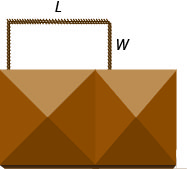Step 3. Name what we are looking for. Let L= the length of the fenced yard. W= the width of the fenced yard Step 4. Translate into a system of equations. One length and two widths equal 125.The length will be 5 feet more than four times the width.The system is: Step 5. Solve the system of equations by substitution.Substitute L = 4W + 5 into the first equation, then solve for W.Substitute 20 for W in the second equation, then solve for L.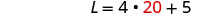Step 6. Check the answer in the problem. $$\begin{array}{rll} 20+28+20&=&125\checkmark \\ 85 &=&4\cdot 20 + 5\checkmark\end{array}$$ Step 7. Answer the equation. The length is 85 feet and the width is 20 feet.
##### Exercise $$\PageIndex{20}$$

Translate to a system of equations and then solve:

Mario wants to put a rectangular fence around the pool in his backyard. Since one side is adjacent to the house, he will only need to fence three sides. There are two long sides and the one shorter side is parallel to the house. He needs 155 feet of fencing to enclose the pool. The length of the long side is 10 feet less than twice the width. Find the length and width of the pool area to be enclosed.

The length is 60 feet and the width is 35 feet.

##### Exercise $$\PageIndex{21}$$

Translate to a system of equations and then solve:

Alexis wants to build a rectangular dog run in her yard adjacent to her neighbor’s fence. She will use 136 feet of fencing to completely enclose the rectangular dog run. The length of the dog run along the neighbor’s fence will be 16 feet less than twice the width. Find the length and width of the dog run.

The length is 60 feet and the width is 38 feet.

## Solve Uniform Motion Applications

We used a table to organize the information in uniform motion problems when we introduced them earlier. We’ll continue using the table here. The basic equation was D = rt where D is the distance traveled, r is the rate, and t is the time.

Our first example of a uniform motion application will be for a situation similar to some we have already seen, but now we can use two variables and two equations.

##### Exercise $$\PageIndex{22}$$

Translate to a system of equations and then solve:

Joni left St. Louis on the interstate, driving west towards Denver at a speed of 65 miles per hour. Half an hour later, Kelly left St. Louis on the same route as Joni, driving 78 miles per hour. How long will it take Kelly to catch up to Joni?

A diagram is useful in helping us visualize the situation.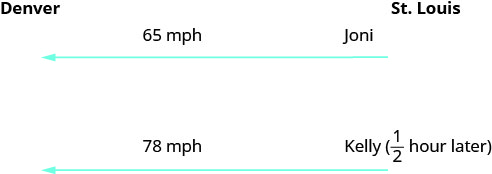Identify and name what we are looking for. A chart will help us organize the data. We know the rates of both Joni and Kelly, and so we enter them in the chart. We are looking for the length of time Kelly, k, and Joni, j, will each drive. Since D=r·t we can fill in the Distance column.Translate into a system of equations. To make the system of equations, we must recognize that Kelly and Joni will drive the same distance. So, 65j=78k. Also, since Kelly left later, her time will be 1212 hour less than Joni’s time. So, k=j−12. Now we have the system.Solve the system of equations by substitution.Substitute k=j−12 into the second equation, then solve for j.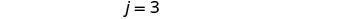To find Kelly’s time, substitute j = 3 into the first equation, then solve for k.Check the answer in the problem.   Joni 3 hours (65 mph) = 195 miles.   Kelly $$2\frac{1}{2}$$ hours (78 mph) = 195 miles.   Yes, they will have traveled the same distance when they meet. Answer the question. Kelly will catch up to Joni in $$2\frac{1}{2}$$ hours. By then, Joni will have traveled 3 hours.
##### Exercise $$\PageIndex{23}$$

Translate to a system of equations and then solve: Mitchell left Detroit on the interstate driving south towards Orlando at a speed of 60 miles per hour. Clark left Detroit 1 hour later traveling at a speed of 75 miles per hour, following the same route as Mitchell. How long will it take Clark to catch Mitchell?

It will take Clark 4 hours to catch Mitchell.

##### Exercise $$\PageIndex{24}$$

Translate to a system of equations and then solve: Charlie left his mother’s house traveling at an average speed of 36 miles per hour. His sister Sally left 15 minutes (1/4 hour) later traveling the same route at an average speed of 42 miles per hour. How long before Sally catches up to Charlie?

It will take Sally $$1\frac{1}{2}$$ hours to catch up to Charlie.

Many real-world applications of uniform motion arise because of the effects of currents—of water or air—on the actual speed of a vehicle. Cross-country airplane flights in the United States generally take longer going west than going east because of the prevailing wind currents.

Let’s take a look at a boat traveling on a river. Depending on which way the boat is going, the current of the water is either slowing it down or speeding it up.

Figure $$\PageIndex{1}$$ and Figure $$\PageIndex{2}$$ show how a river current affects the speed at which a boat is actually traveling. We’ll call the speed of the boat in still water b and the speed of the river current c.

In Figure $$\PageIndex{1}$$ the boat is going downstream, in the same direction as the river current. The current helps push the boat, so the boat’s actual speed is faster than its speed in still water. The actual speed at which the boat is moving is b + c.Figure $$\PageIndex{1}$$

In Figure $$\PageIndex{2}$$ the boat is going upstream, opposite to the river current. The current is going against the boat, so the boat’s actual speed is slower than its speed in still water. The actual speed of the boat is b−c.Figure $$\PageIndex{2}$$

We’ll put some numbers to this situation in Exercise $$\PageIndex{25}$$.

##### Exercise $$\PageIndex{25}$$

Translate to a system of equations and then solve:

A river cruise ship sailed 60 miles downstream for 4 hours and then took 5 hours sailing upstream to return to the dock. Find the speed of the ship in still water and the speed of the river current.

This is a uniform motion problem and a picture will help us visualize the situation.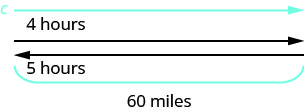Identify what we are looking for. We are looking for the speed of the ship in still water and the speed of the current. Name what we are looking for. Let s=s= the rate of the ship in still water. c=c= the rate of the current A chart will help us organize the information. The ship goes downstream and then upstream. Going downstream, the current helps the ship; therefore, the ship’s actual rate is s + c. Going upstream, the current slows the ship; therefore, the actual rate is s − c.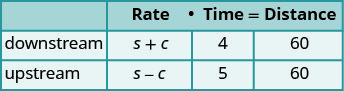Downstream it takes 4 hours. Upstream it takes 5 hours. Each way the distance is 60 miles. Translate into a system of equations. Since rate times time is distance, we can write the system of equations.Solve the system of equations. Distribute to put both equations in standard form, then solve by elimination.Multiply the top equation by 5 and the bottom equation by 4. Add the equations, then solve for s.Substitute s = 13.5 into one of the original equations.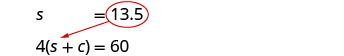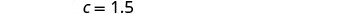Check the answer in the problem.  The downstream rate would be   13.5 + 1.5 = 15 mph.  In 4 hours the ship would travel     15 · 4 = 60 miles.  The upstream rate would be   13.5 − 1.5 = 12 mph.  In 5 hours the ship would travel     12 · 5 = 60 miles. Answer the question. The rate of the ship is 13.5 mph and the rate of the current is 1.5 mph.
##### Exercise $$\PageIndex{26}$$

Translate to a system of equations and then solve: A Mississippi river boat cruise sailed 120 miles upstream for 12 hours and then took 10 hours to return to the dock. Find the speed of the river boat in still water and the speed of the river current.

The rate of the boat is 11 mph and the rate of the current is 1 mph.

##### Exercise $$\PageIndex{27}$$

Translate to a system of equations and then solve: Jason paddled his canoe 24 miles upstream for 4 hours. It took him 3 hours to paddle back. Find the speed of the canoe in still water and the speed of the river current.

The speed of the canoe is 7 mph and the speed of the current is 1 mph.

Wind currents affect airplane speeds in the same way as water currents affect boat speeds. We’ll see this in Exercise $$\PageIndex{28}$$. A wind current in the same direction as the plane is flying is called a tailwind. A wind current blowing against the direction of the plane is called a headwind.

##### Exercise $$\PageIndex{28}$$

Translate to a system of equations and then solve:

A private jet can fly 1095 miles in three hours with a tailwind but only 987 miles in three hours into a headwind. Find the speed of the jet in still air and the speed of the wind.

This is a uniform motion problem and a picture will help us visualize.Identify what we are looking for. We are looking for the speed of the jet in still air and the speed of the wind. Name what we are looking for. Let j= the speed of the jet in still air. w= the speed of the wind A chart will help us organize the information. The jet makes two trips-one in a tailwind and one in a headwind. In a tailwind, the wind helps the jet and so the rate is j + w. In a headwind, the wind slows the jet and so the rate is j − w.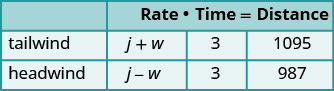Each trip takes 3 hours. In a tailwind the jet flies 1095 miles. In a headwind the jet flies 987 miles. Translate into a system of equations. Since rate times time is distance, we get the system of equations.Solve the system of equations. Distribute, then solve by elimination.Add, and solve for j. Substitute j = 347 into one of the original equations, then solve for w.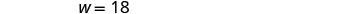Check the answer in the problem.  With the tailwind, the actual rate of the  jet would be    347 + 18 = 365 mph.  In 3 hours the jet would travel     365 · 3 = 1095 miles.  Going into the headwind, the jet’s actual  rate would be    347 − 18 = 329 mph.  In 3 hours the jet would travel     329 · 3 = 987 miles. Answer the question. The rate of the jet is 347 mph and the rate of the wind is 18 mph.
##### Exercise $$\PageIndex{29}$$

Translate to a system of equations and then solve: A small jet can fly 1,325 miles in 5 hours with a tailwind but only 1025 miles in 5 hours into a headwind. Find the speed of the jet in still air and the speed of the wind.

The speed of the jet is 235 mph and the speed of the wind is 30 mph.

##### Exercise $$\PageIndex{30}$$

Translate to a system of equations and then solve: A commercial jet can fly 1728 miles in 4 hours with a tailwind but only 1536 miles in 4 hours into a headwind. Find the speed of the jet in still air and the speed of the wind.

Two angles are complementary if the sum of the measures of their angles is $$90$$ degrees.
Two angles are supplementary if the sum of the measures of their angles is $$180$$ degrees.